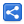# JMX quick start

[Last Updated: May 18, 2017]

Since release 5.0, Java Management Extensions (JMX) technology is a standard part of JSE platform. JMX provides a simple, standard way of dynamically monitoring and managing application resources. In this tutorial we will learn what we can do with JMX with an example.

## Writing Standard MBeans

We will write our application as usual, but by implementing a special interface (called MBean interface), we will expose certain attributes to be managed by a JMX console application (e.g. JConsole) externally.

```public interface CalculatorMBean {

void setDecimalPlaces(int decimalPlaces);

int getDecimalPlaces();
}```

Following is the implementation of MBean.

```public class Calculator implements CalculatorMBean {
private int decimalPlaces = 2;

public double add(double d1, double d2) {
BigDecimal bd1 = new BigDecimal(d1);
BigDecimal bd2 = new BigDecimal(d2);

return sum.setScale(decimalPlaces, RoundingMode.HALF_UP)
.doubleValue();
}

public void setDecimalPlaces(int decimalPlaces) {
this.decimalPlaces = decimalPlaces;
}

public int getDecimalPlaces() {
return decimalPlaces;
}
}```

By convention, a MBean interface takes the name of the Java class that implements it, with the suffix MBean added.

## Application logic and JMX agent

Following main class writes a command line application which asks user to enter two number whose sum is returned after rounding the result according to the 'decimalPlaces' attribute.

Our main class also registers the Calculator MBean to MBeanServer. That means our main class exposes the application as a JMX agent so that an external JMX console (e.g. JConsole) can connect and manage the MBean attributes.

```public class AppMain {

public static void main(String[] args) throws Exception {

Calculator calculator = new Calculator();
registerWithJmxAgent(calculator);
startConsoleApp(calculator);
}

private static void startConsoleApp(Calculator calculator) {
Scanner scanner = new Scanner(System.in);

while (true) {
System.out.println("-----------------");
String input1 = getUserInput(scanner, "enter first number");
double d1 = toDouble(input1);

String input2 = getUserInput(scanner, "enter second number");
double d2 = toDouble(input2);

System.out.printf("sum = %s (rounded to %s decimal places)%n",
sum, calculator.getDecimalPlaces());
}
}

private static double toDouble(String input) {
try {
return Double.parseDouble(input);
} catch (NumberFormatException e) {
System.out.println("Not a valid number, defaulting to 0");
return 0;
}
}

private static void registerWithJmxAgent(Calculator calculator) throws Exception {
MBeanServer mbs = ManagementFactory.getPlatformMBeanServer();
ObjectName name = new ObjectName("com.logicbig.example:type=calculator");
mbs.registerMBean(calculator, name);
}

public static String getUserInput(Scanner scanner, String msg) {
System.out.print(msg + ">");
String s = scanner.nextLine();
if ("exit".equals(s)) {
System.exit(0);
}
return s;
}
}```

#### Running the application

```mvn -q compile exec:java -Dexec.mainClass="com.logicbig.example.AppMain"
```

Entering the two numbers:

```-----------------
enter first number>1.322
enter second number>4.333
sum = 5.66 (rounded to 2 decimal places)
-----------------
```

## Managing via JConsole

```C:\>jconsole
```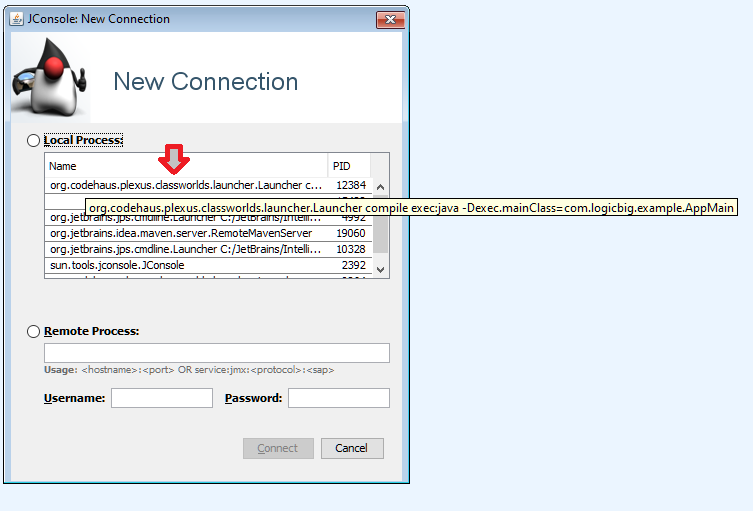This is how we alter the MBean attributes during runtime.We changed the 'decimalPlaces' attribute to 1 decimal.

Now result sum will be of one decimal place.

```-----------------
enter first number>1.322
enter second number>4.333
sum = 5.7 (rounded to 1 decimal places)
-----------------
```

## Example video

The step by step example video:

## Example Project

Dependencies and Technologies Used:

• JDK 1.8
• Maven 3.3.9

 Jmx Quick Start Example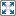Select All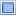Download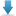• jmx-quick-start
• src
• main
• java
• com
• logicbig
• example
• CalculatorMBean.java

 Share